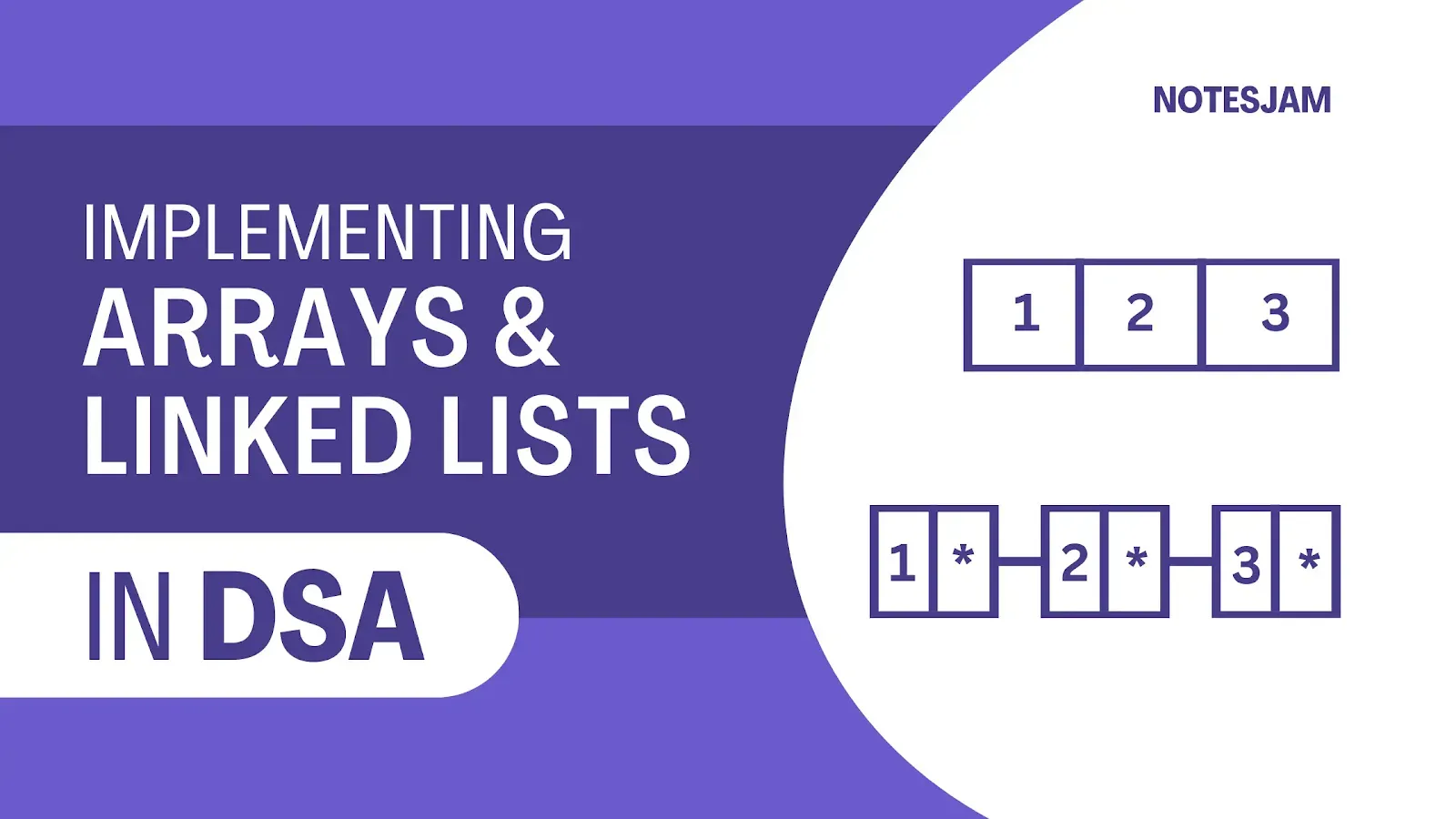Save important notes and articles from internet using arusaquotes app.

As a programmer, you will often need to store and manipulate large amounts of data in your programs. Two common ways of doing this are through the use of arrays and linked lists.

While both of these data structures can be used to store data, they differ in their implementation and usage.

## Implementing Arrays

An array is a collection of data that is stored in contiguous memory locations. Each element in the array is accessed using its index, which is an integer value that represents the position of the element in the array.

To implement an array in your program, you can follow these steps:

Declare an array: To declare an array, you need to specify its data type and the number of elements it will contain. For example, to declare an array of integers with 10 elements, you can use the following code:

````int myArray;`
```

Initialize the array: After declaring the array, you can initialize its elements with values. You can either do this at the time of declaration or later in your code.

To initialize the elements of the array at the time of declaration, you can use the following code:

````int myArray = {1, 2, 3, 4, 5, 6, 7, 8, 9, 10};`
```

Access the elements of the array: To access the elements of the array, you can use their index. The index of the first element in the array is always 0. For example, to access the first element of the array, you can use the following code:

````int firstElement = myArray;`
```

A linked list is a data structure that consists of a sequence of nodes, where each node contains a value and a pointer to the next node in the list.

The first node in the list is called the head, and the last node is called the tail. To implement a linked list in your program, you can follow these steps:

Define the node structure: To define a node structure, you need to create a struct that contains the data value and a pointer to the next node in the list.

For example, to define a node structure for a linked list of integers, you can use the following code:

``````struct Node {
int data;
Node* next;
};```
```

Create the head node: To create the head node, you need to allocate memory for the node structure and initialize its data value and next pointer.

For example, to create the head node for a linked list of integers with a value of 1, you can use the following code:

``````Node* head = new Node;
```

Add nodes to the list: To add a node to the list, you need to allocate memory for the node structure, initialize its data value, and set its next pointer to the appropriate node in the list.

For example, to add a node with a value of 2 after the head node, you can use the following code:

``````Node* newNode = new Node;
newNode->data = 2;
```

Traverse the list: To traverse the list, you need to start at the head node and follow the next pointers until you reach the end of the list.

For example, to traverse a linked list of integers and print its values, you can use the following code:

``````Node* currentNode = head;
while (currentNode != nullptr) {
std::cout << currentNode->data << " ";
currentNode = currentNode->next;
}```
```

## Conclusion

Arrays and linked lists are two common data structures that can be used to store and manipulate data in your programs.

While arrays are simpler to implement, they have a fixed size and can be inefficient when adding or removing elements. Linked lists, on the other hand, can dynamically grow and shrink in size, but require more memory allocation and deallocation.

By understanding the steps involved in implementing arrays and linked lists, you can choose the appropriate data structure for your program and efficiently manage your data.